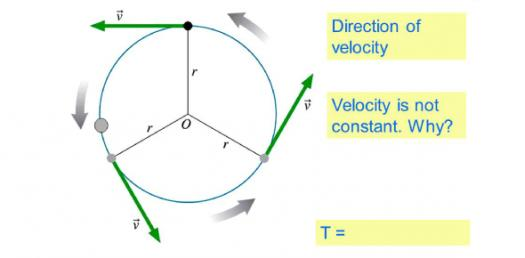# AP Physics: Trivia Quiz On Circular Motion And Gravitation!

Approved & Edited by ProProfs Editorial Team
At ProProfs Quizzes, our dedicated in-house team of experts takes pride in their work. With a sharp eye for detail, they meticulously review each quiz. This ensures that every quiz, taken by over 100 million users, meets our standards of accuracy, clarity, and engagement.
| Written by Sfduke
S
Sfduke
Community Contributor
Quizzes Created: 57 | Total Attempts: 8,166
Questions: 5 | Attempts: 223Settings.

• 1.

### When calculating the gravitational force between two extended bodies, you should measure the distance

• A.

From the closest points on each body.

• B.

From the most distant points on each body.

• C.

From the center of each body.

• D.

From the center of one body to the closest point on the other body.

C. From the center of each body.
Explanation
When calculating the gravitational force between two extended bodies, it is important to measure the distance from the center of each body. This is because the center of mass of each body is the point at which the gravitational force acts as if all the mass is concentrated. By measuring the distance from the center of each body, we can accurately determine the gravitational force between them.

Rate this question:

• 2.

### What is the centripetal force that holds planets in orbit?

• A.

Inertia

• B.

Gravitational force

• C.

Planetary force

• D.

Kepler’s force

B. Gravitational force
Explanation
The centripetal force that holds planets in orbit is the gravitational force. This force is exerted by the sun or another massive object towards the center of the orbit, causing the planets to continuously move in a curved path. The gravitational force between the planet and the sun keeps the planet in orbit and prevents it from moving away or falling into the sun. This force is proportional to the masses of the objects and inversely proportional to the square of the distance between them, as described by Newton's law of universal gravitation.

Rate this question:

• 3.

### The force that Earth exerts on the moon

• A.

Is greater than the force the moon exerts on Earth.

• B.

Is less than the force the moon exerts on Earth.

• C.

Is equal in magnitude to the force the moon exerts on Earth.

• D.

Causes tides.

C. Is equal in magnitude to the force the moon exerts on Earth.
Explanation
The force that Earth exerts on the moon is equal in magnitude to the force the moon exerts on Earth because of Newton's third law of motion, which states that for every action, there is an equal and opposite reaction. The gravitational force between two objects depends on their masses and the distance between them. Since the mass of the Earth and the moon are different, the force of gravity between them is also different. However, according to Newton's third law, the forces they exert on each other are equal in magnitude but opposite in direction. Therefore, the force that Earth exerts on the moon is equal to the force the moon exerts on Earth.

Rate this question:

• 4.

### How does the gravitational force between two objects change if the distance between the objects doubles?

• A.

The force decreases by a factor of 4.

• B.

The force decreases by a factor of 2.

• C.

The force increases by a factor of 2.

• D.

The force increases by a factor of 4.

A. The force decreases by a factor of 4.
Explanation
The gravitational force between two objects is inversely proportional to the square of the distance between them. This means that if the distance between the objects doubles, the force will decrease by a factor of 4. This is because when the distance doubles, the denominator in the equation for gravitational force becomes 4 times larger, resulting in a force that is 1/4 of the original force.

Rate this question:

• 5.

### The law of universal gravitation states that

• A.

Two objects always exert gravitational forces on each other.

• B.

The force of gravity depends on the mass and the distance between the two objects.

• C.

All objects fall at different rates on earth.

• D.

Both a and b.Back to top